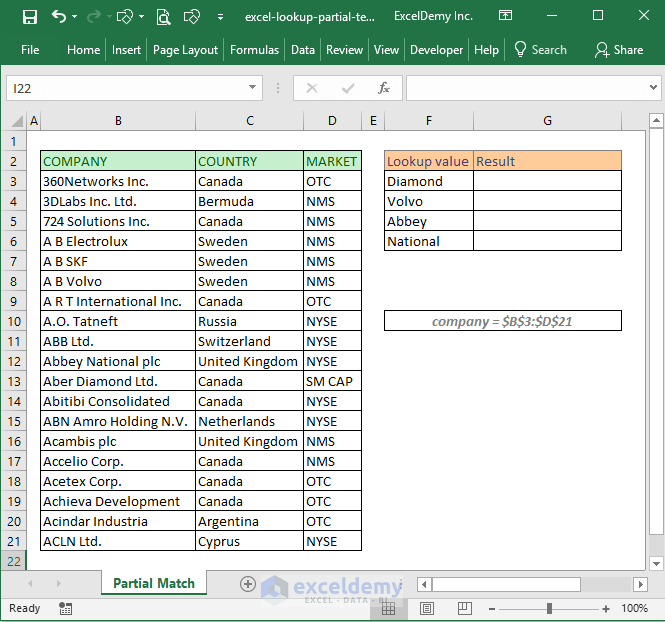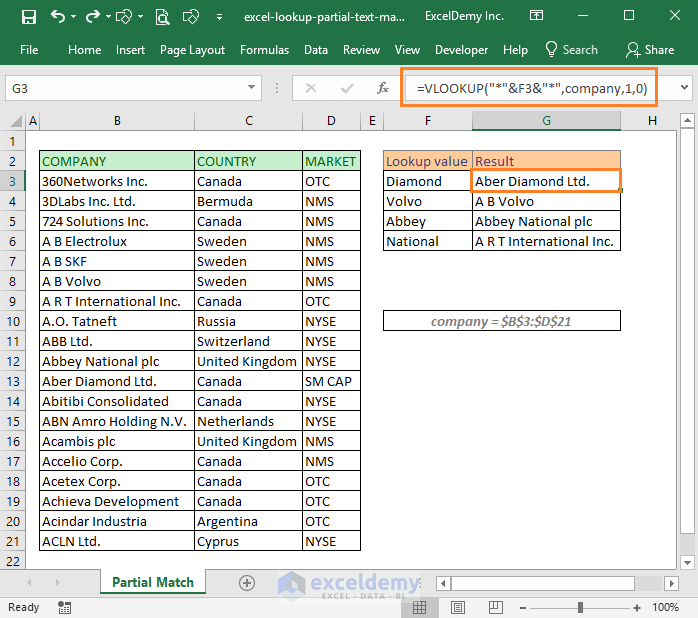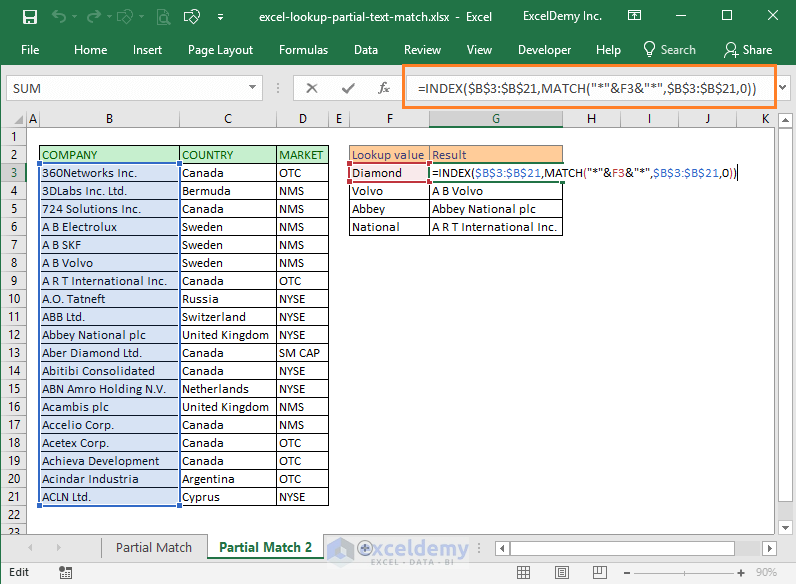# Using Excel to Lookup Partial Text Match [2 Ways]

In this article, I will show you how to use Excel to lookup for partial text match. For this, we can use VLOOKUP or INDEX MATCH functions combo.

In the following image, you are seeing some company names, their origin country, and market category. We want to return full company name using a partial match of the name.Say, if we look up with Diamond, we want that full company name “Aber Diamond Ltd.” will return. Or if we search with Abbey, we want “Abbey National plc” will be returned.

## Excel lookup partial text match (using VLOOKUP)

In cell G3, we use this formula: =VLOOKUP(“*”&F3&”*”,company,1,0)

And then copy paste the formula for other cells in the column. And this is what we get.How does this formula work?

This is a very simple formula. Only the complex part of this formula is this part: “*”&F3&”*”

If we use the value of cell F3 as the replacement, it will look like “*Diamond*”.

This character (*) is actually Excel’s wildcard character and it is replaced by any characters.

So, this text “*Diamond*” means: it is any text that has Diamond, also having any characters before and after Diamond.

We are using “*Diamond*” as VLOOKUP function’s lookup_value. So, VLOOKUP will search for any text in the lookup_array that contains Diamond text.

I hope this explanation clears the idea how this formula actually works.

## Excel lookup partial text match (using INDEX Match combo)

This time, we shall use this formula in cell G3: =INDEX(\$B\$3:\$B\$21,MATCH(“*”&F3&”*”,\$B\$3:\$B\$21,0))

And we get the same results when copy and paste the formula for other cells in the column.

Check out the following page.These are my two ways of using Excel to lookup partial text match. If you know any other technique to do this, let me know in the comment box.

#### Kawser

Hello! Welcome to my Excel blog! It took me some time to be a fan of Excel. But now I am a die-hard fan of MS Excel. I learn new ways of doing things with Excel and share here. Not only how to guide on Excel, but you will get also topics on Finance, Statistics, Data Analysis, and BI. Stay tuned!

Sonu Singh August 22, 2018 at 11:32 AM

Thanks Sir,

It’s Very Good Idea.

Bertrand James November 25, 2019 at 10:20 PM

I,HAVE LEARNED SOMETHING NEW

November 26, 2019 at 9:16 AM